﻿ 先进战斗机过失速机动大气数据融合估计方法Download PDF文章快速检索 高级检索

1. 中国航空工业成都飞机设计研究所, 成都 610091;
2. 南京航空航天大学 自动化学院, 南京 210016

Air data fusion and estimation method for advanced aircrafts in post-stall maneuver
YANG Zhaoxu1, GUO Yi1, LEI Tingwan1, LI Rongbing2
1. AVIC Chengdu Aircraft Design and Research Institute, Chengdu 610091, China;
2. College of Automation Engineering, Nanjing University of Aeronautics and Astronautics, Nanjing 210016, China
Abstract: Post-stall maneuver capability is an important feature of advanced aircrafts, and the extension of flight envelope exceeded the measurement range of traditional air data systems. air data including angle of attack, angle of sideslip, total and static pressure are the essential factors for the control of advanced fighters in post-stall maneuver. An air data fusion and estimation method was proposed and validated based on a thrust vector technology test. The first step is to calculate air data by blending vectors of wind speed, ground speed, and navigation parameters such as attitude and rotation rate. A kind of deep neural network with multiple hidden layers and better feature expression and ability to model complex mapping was designed, trained and employed to fit the calculated angle of attack errors with strong nonlinearity and hysteresis, which were due to the unsteady flow around the aircraft and nonlinear relationship among the flight state parameters. Simulation and flight data shown that the proposed method can complete the estimation of air data even at high angle of attack maneuver and achieve angle of attack parameter with an error no more than 2.3°. The estimated air data can be provided to the flight control system as reliable state feedback.
Keywords: post-stall maneuver    high angle of atteck    air data    wind estimation    inertial navigation    deep neural network

1 方法介绍 1.1 过失速机动飞行试验的大气数据特性分析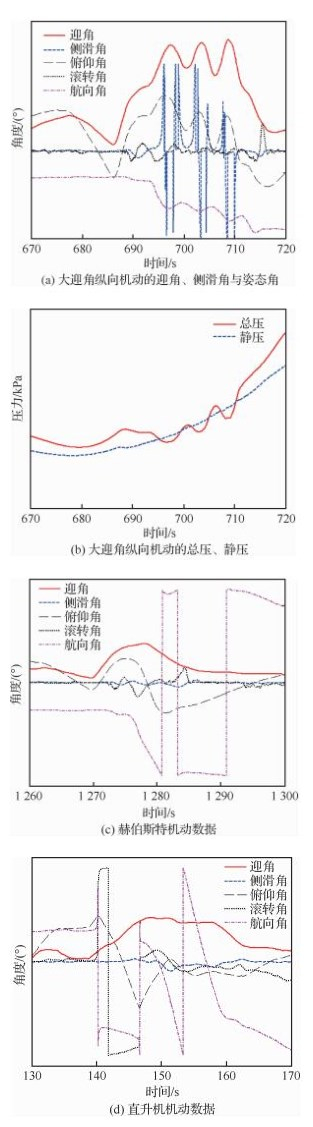图 1 过失速机动迎角、侧滑角、总静压、姿态角曲线 Fig. 1 Variation curves of measured AOA, AOS, total and static pressure, attitude angles in post-stall maneuver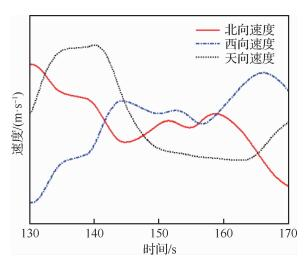图 2 直升机机动的三维速度曲线 Fig. 2 3D speed curves in helicopter maneuver

1.2 过失速机动大气数据融合估计方案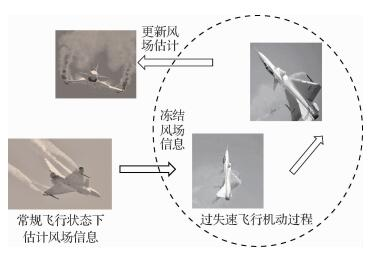图 3 飞行状态转换过程示意图 Fig. 3 Schematic diagram of flight state transition process

1) 在常规飞行状态，基于机载惯性/卫星组合导航系统和大气数据系统的输出信息，估计风场参数。

2) 进入过失速机动后，停止风场参数的估计，在过失速机动的飞行时间和空间范围内，冻结风场参数。

3) 利用冻结的风场参数、机载惯性/卫星导航系统的导航参数，进行大气参数估计，包括迎角与侧滑角的计算。

4) 基于飞行数据，训练深度神经网络，利用深度神经网络对迎角复杂非线性误差进行补偿。

5) 在迎角、侧滑角基础上，进一步计算过失速机动状态下的真空速、马赫数、静温、总压、静压等大气参数。

6) 过失速机动过程结束、恢复常规飞行状态后，大气数据系统恢复有效性，开始风场信息的估计与更新。

2 基于风场模型的风场信息滤波

 $\mathit{\boldsymbol{V}}_{\rm{W}}^{\rm{n}} = \mathit{\boldsymbol{C}}_{\rm{b}}^{\rm{n}}{\kern 1pt} \mathit{\boldsymbol{V}}_{\rm{W}}^{\rm{b}} = \mathit{\boldsymbol{V}}_{\rm{G}}^{\rm{n}} - \mathit{\boldsymbol{C}}_{\rm{b}}^{\rm{n}}{\kern 1pt} \mathit{\boldsymbol{V}}_{\rm{T}}^{\rm{b}}$ （1）

 $\mathit{\boldsymbol{V}}_{\rm{G}}^{\rm{n}} = \mathit{\boldsymbol{V}}_{{\rm{G, 0}}}^{\rm{n}} + \mathit{\boldsymbol{\omega }} \times \mathit{\boldsymbol{R}}$ （2）

 $\mathit{\boldsymbol{V}}_{\rm{T}}^{\rm{b}} = \left[ {\begin{array}{*{20}{l}} {v_{{\rm{T, }}x}^{\rm{b}}}\\ {v_{{\rm{T, }}y}^{\rm{b}}}\\ {v_{{\rm{T, }}z}^{\rm{b}}} \end{array}} \right] = \left[ {\begin{array}{*{20}{c}} {{V_{\rm{T}}}{\rm{cos}}{\kern 1pt} {\kern 1pt} \beta {\kern 1pt} {\kern 1pt} {\rm{cos}}{\kern 1pt} {\kern 1pt} {\kern 1pt} \alpha }\\ {{V_{\rm{T}}}{\rm{sin}}{\kern 1pt} {\kern 1pt} \beta }\\ {{V_{\rm{T}}}{\rm{cos}}{\kern 1pt} {\kern 1pt} \beta {\kern 1pt} {\kern 1pt} {\rm{sin}}{\kern 1pt} {\kern 1pt} {\kern 1pt} \alpha } \end{array}} \right]$ （3）

 $\mathit{\boldsymbol{V}}_{\rm{W}}^{\rm{n}} = \mathit{\boldsymbol{V}}_{{\rm{W, c}}}^{\rm{n}} + \mathit{\boldsymbol{V}}_{{\rm{W, t}}}^{\rm{n}}$ （4）

3 基于冻结风场和导航数据的大气数据计算模型

 $\mathit{\boldsymbol{V}}_{\rm{T}}^{\rm{b}} = \mathit{\boldsymbol{C}}_{\rm{n}}^{\rm{b}}(\mathit{\boldsymbol{V}}_{\rm{G}}^{\rm{n}} - \mathit{\boldsymbol{V}}_{\rm{W}}^{\rm{n}})$ （5）
 $\alpha = {\rm{arctan}}\frac{{V_{{\rm{T}}, z}^{\rm{b}}}}{{V_{{\rm{T}}, y}^{\rm{b}}}}$ （6）
 $\beta = {\rm{arctan}}\frac{{V_{{\rm{T}}, x}^{\rm{b}}}}{{\sqrt {{{(V_{{\rm{T}}, y}^{\rm{b}})}^2} + {{(V_{{\rm{T}}, z}^{\rm{b}})}^2}} }}$ （7）
 ${V_{\rm{T}}} = \sqrt {{{(V_{{\rm{T}}, x}^{\rm{b}})}^2} + {{(V_{{\rm{T}}, y}^{\rm{b}})}^2} + {{(V_{{\rm{T}}, z}^{\rm{b}})}^2}}$ （8）
 ${H_{\rm{p}}} = {H_{\rm{I}}} + {H_{{\rm{bc}}}}$ （9）
 ${P_{\rm{s}}} = {P_{\rm{b}}}{\rm{exp}}\left( {\frac{{ - {g_{\rm{n}}}}}{{R{T_{\rm{b}}}}}({H_{\rm{p}}} - {H_{\rm{b}}})} \right)$ （10）
 ${T_{\rm{s}}} = T({H_{\rm{p}}}) + {T_{{\rm{bc}}}}$ （11）
 ${T_{\rm{t}}} = {T_{\rm{s}}}(1 + 0.2M{a^2})$ （12）
 $Ma = \frac{{{V_{\rm{T}}}}}{{72.164{\kern 1pt} {\kern 1pt} {\kern 1pt} 86\sqrt {{T_{\rm{s}}}} }}$ （13）
 ${P_{\rm{t}}} = \left\{ {\begin{array}{*{20}{l}} {\frac{{{P_{\rm{s}}}}}{{{{(1 + 0.2M{a^2})}^{ - 3.5}}}}}&{Ma \le 1}\\ {{P_{\rm{s}}} \cdot 1.2M{a^2} \cdot {{\left( {\frac{{7.2M{a^2}}}{{7M{a^2} - 1}}} \right)}^{2.5}}}&{Ma > 1} \end{array}} \right.$ （14）

4 基于深度多层神经网络的迎角误差补偿方法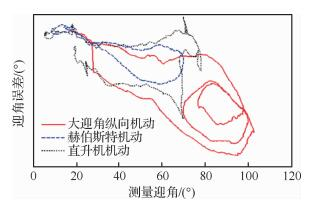图 4 计算迎角的误差与测量迎角的对应曲线 Fig. 4 Curves of error of calculated AOA with change of measured AOA

1) 训练数据集合构建

2) 深度神经网络层数和节点数迭代训练

 第1隐层节点数 第2隐层节点数 第3隐层节点数 损失函数 80 30 5 0.36 100 30 5 0.67 80 50 5 0.46 100 50 5 0.24 80 30 10 0.20 100 30 10 0.26 80 50 10 0.31 100 50 10 0.52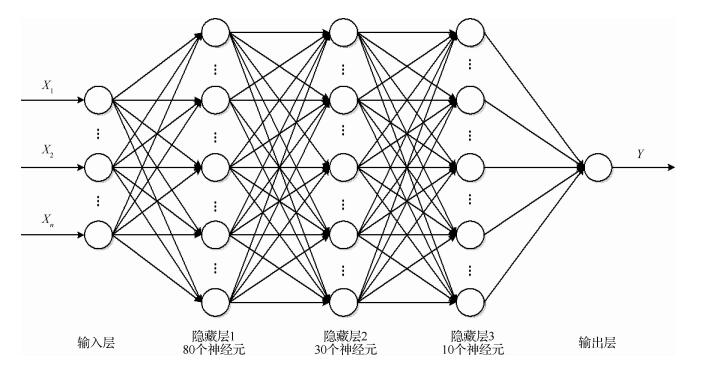图 5 过失速机动融合迎角的偏差修正深度神经网络结构图 Fig. 5 Structural chart of depth neural network for post-stall maneuvering correction of fusion angle of attack
5 过失速机动大气参数融合与补偿方法试验验证

1) 风速估计与风场冻结的误差分析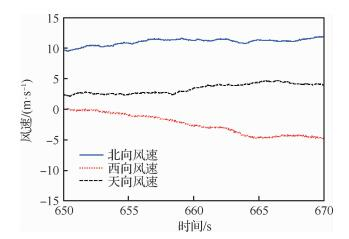图 6 过失速大迎角纵向机动前的风速估计曲线 Fig. 6 Estimated wind speed before post-stall longitudinal maneuver at high AOA

2) 迎角融合与补偿分析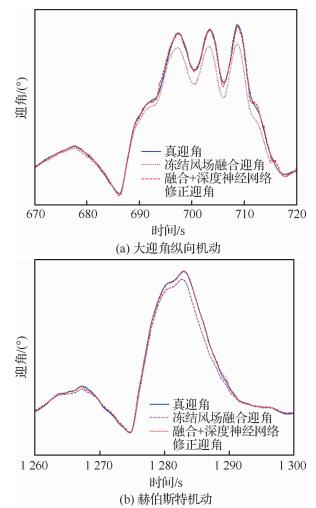图 7 典型过失速机动的融合迎角与真迎角曲线 Fig. 7 Curves of fused AOA and true AOA in typical post-stall maneuvers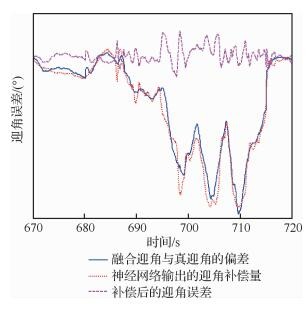图 8 大迎角纵向机动的迎角误差拟合与补偿残差曲线 Fig. 8 Curves of AOA error fitting and residual in longitudinal maneuver with high AOA

 机动类型 融合迎角最大相对误差/(°) 融合迎角+ DNN补偿后的最大相对误差/(°) 融合迎角+ DNN补偿后误差均方差/(°) 大迎角纵向机动 0.13 0.02 0.5 赫伯斯特机动 0.08 0.03 0.4 直升机机动 0.19 0.03 0.4 榔头机动 0.12 0.03 0.5 大迎角360°滚转 0.12 0.02 0.4

3) 总静压与侧滑角的融合估计结果分析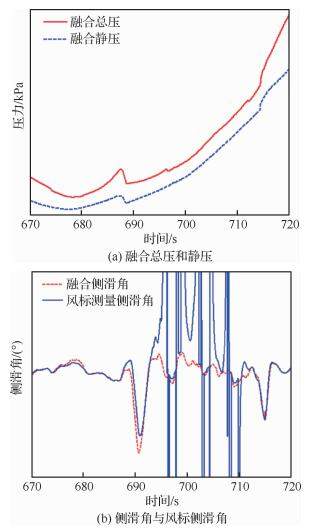图 9 大迎角纵向机动时总压、静压和侧滑角曲线 Fig. 9 Curves of fused total pressure, static pressure and fused AOS in longitudinal maneuver with high AOA
6 结论

1) 过失速机动为瞬态过程，基于过失速机动前的常规飞行状态估计的风场信息，在过失速机动过程中，冻结风场信息在时间连续性和空间关联性方面的假设合理，飞行试验数据支持了上述假设的合理性。

2) 进入过失速机动状态后，基于冻结的风速信息、地速和惯性姿态、导航参数，可以融合得到总压、静压、迎角、侧滑角等大气参数，相对于大气数据测量得到的信息，融合估计得到参数更符合过失速机动物理过程。

3) 以试飞状态风标传感器的真迎角为基准，对直接融合得到的迎角信息的误差进行研究，利用深度神经网络对复杂非线性问题的拟合能力，对迎角偏差进行拟合，表明：通过过失速飞行状态下的融合迎角和补偿，多个典型过失速机动飞行过程的迎角误差优于2.3°。

4) 风标传感器测量的真实迎角依然受到大迎角气动非定常现象的影响，但为了方便进行对比说明，仍然采用该迎角作为比较的基准。后续需要进行气动非定常对机头空速管风标测量误差的定量研究。

  朱纪洪, 张尚敏, 周池军, 等. 飞机超机动状态动力学特征及对控制系统的挑战[J]. 控制理论与应用, 2014, 31(12): 1650-1662. ZHU J H, ZHANG S M, ZHOU C J, et al. Dynamic characteristics and challenges for control system of super-maneuverable aircraft[J]. Control Theory & Applications, 2014, 31(12): 1650-1662. (in Chinese) Cited By in Cnki (17) | Click to display the text  史忠科. 高性能飞机发展对控制理论的挑战[J]. 航空学报, 2015, 36(8): 2717-2734. SHI Z K. Challenge of control theory in the presence of high performance aircraft development[J]. Acta Aeronautica et Astronautica Sinica, 2015, 36(8): 2717-2734. (in Chinese) Cited By in Cnki (12) | Click to display the text  汪清, 钱炜祺, 丁娣. 飞机大迎角非定常气动力建模研究进展[J]. 航空学报, 2016, 37(8): 2331-2347. WANG Q, QIAN W Q, DING D. A review of unsteady aerodynamic modeling of aircraft at high angles of attack[J]. Acta Aeronautica et Astronautica Sinica, 2016, 37(8): 2331-2347. (in Chinese) Cited By in Cnki (16) | Click to display the text  HYDE D, BROWN F, SHWEYK K, et al. High angle of attack static and dynamic modeling methods for flight dynamics loss of control research[C]//2018 AIAA Atmospheric Flight Mechanics Conference. Reston: AIAA, 2018.  张婉鑫, 朱纪洪. 大迎角非定常气动参数辨识研究[J]. 清华大学学报(自然科学版), 2017, 57(7): 673-679. ZhANG W X, ZHU J H. Unsteady aerodynamic identification of aircraft at high angle of attack[J]. Journal Tsinghua University (Science and Technology), 2017, 57(7): 673-679. (in Chinese) Cited By in Cnki (2) | Click to display the text  SHI J P, LV Y X, QU X B, et al. A coordinated control method of thrust vector and aerodynamic surfaces based on control allocation technology[C]//2018 18th International Conference on Control, Automation and Systems, 2018.  汤伟, 黄勇, 傅澔. 推力矢量对飞机大迎角动态气动特性的影响[J]. 航空学报, 2018, 39(4): 121648. TANG W, HUANG Y, FU H. Effect of thrust vector on dynamic aerodynamic characteristics of aircraft at high angle of attack[J]. Acta Aeronautica et Astronatica Sinica, 2018, 39(4): 121648. (in Chinese) Cited By in Cnki (5) | Click to display the text  HARRIS J. F-35 flight control law design, development and verification[C]//2018 Aviation Technology, Integration, and Operations Conference. Reston: AIAA, 2018.  陈海, 何开锋, 钱炜褀, 等. 基于扩展L1自适应的战斗机大迎角非线性控制[J]. 控制与决策, 2017, 32(8): 1403-1408. CHEN H, HE K F, QIAN W Q, et al. L1 adaptive augmentation for high angle of attack nonlinear control of fighter[J]. Control and Decision, 2017, 32(8): 1403-1408. (in Chinese) Cited By in Cnki (4) | Click to display the text  叶辉, 吴庆宪, 陈谋. 非定常条件下大迎角机动控制[J]. 哈尔滨工业大学学报, 2016, 48(4): 84-90. YE H, WU Q X, CHEN M. Control of high angle of attack maneuver under unsteady aerodynamic condition[J]. Journal of Harbin Institute of Technology, 2016, 48(4): 84-90. (in Chinese) Cited By in Cnki (1) | Click to display the text  陆辰, 李荣冰, 刘建业, 等. 气动模型及导航信息辅助的大气参数估计方法[J]. 控制与决策, 2018, 33(3): 491-496. LU C, LI R B, LIU J Y, et al. Air data estimation method aided by aerodynamic model and navigation information[J]. Control and Decision, 2018, 33(3): 491-496. (in Chinese) Cited By in Cnki (3) | Click to display the text  NEBULA F, PALUMBO R, MORANI G. Virtual air data: A fault-tolerant approach against ADS failures[C]//AIAA Infotech at Aerospace Conference, Reston: AIAA, 2013: 1-14.  LANGELAAN J W, SPLETZER J, MONTELLA C, et al. Wind field estimation for autonomous dynamic soaring[C]//2012 IEEE International Conference on Robotics and Automation. Piscataway: IEEE Press, 2012: 16-22.  LI R B, LU C, LIU J Y, et al. Air data estimation algorithm under unknown wind based on information fusion[J]. Journal of Aerospace Engineering, 2018, 31(5): 04018072. Click to display the text  焦李成, 杨淑媛, 刘芳, 等. 神经网络七十年:回顾与展望[J]. 计算机学报, 2016, 39(8): 1697-1716. JIAO L C, YANG S Y, LIU F, et al. Seventy years beyond neural networks:Retrospect and prospect[J]. Chinese Journal of Computer, 2016, 39(8): 1697-1716. (in Chinese) Cited By in Cnki (550) | Click to display the text  曾毅, 刘成林, 谭铁牛. 类脑只能研究的回顾与展望[J]. 计算机学报, 2016, 39(1): 212-222. ZENG Y, LIU C L, TAN T N. Retrospect and outlook of brain-inspired intelligence research[J]. Chinese Journal of Computer, 2016, 39(1): 212-222. (in Chinese) Cited By in Cnki | Click to display the text  陈建廷, 向阳. 深度神经网络训练中梯度不稳定线型研究综述[J]. 软件学报, 2018, 29(7): 2071-2091. CHEN J T, XIANG Y. Survey of unstable gradients in deep neural network training[J]. Journal of Software, 2018, 29(7): 2071-2091. (in Chinese) Cited By in Cnki (35) | Click to display the text  高晓光, 李新宇, 岳勐琪, 等. 基于深度学习的地空导弹发射区拟合算法研究[J]. 航空学报, 2019, 40(9): 322858. GAO X G, LI X Y, YUE M Q, et al. Fitting algorithm of ground-to-air missile launching area based on deep learning[J]. Acta Aeronautica et Astronautica Sinica, 2019, 40(9): 322858. (in Chinese) Cited By in Cnki | Click to display the text  朱虎明, 李佩, 焦李成, 等. 深度神经网络并行化研究综述[J]. 计算机学报, 2018, 41(8): 1862-1881. ZHU H M, LI P, JIAO L C, et al. Review of parallel deep neural network[J]. Chinese Journal of Computer, 2018, 41(8): 1862-1881. (in Chinese) Cited By in Cnki (42) | Click to display the text
http://dx.doi.org/10.7527/S1000-6893.2019.23456

0

#### 文章信息

YANG Zhaoxu, GUO Yi, LEI Tingwan, LI Rongbing

Air data fusion and estimation method for advanced aircrafts in post-stall maneuver

Acta Aeronautica et Astronautica Sinica, 2020, 41(6): 523456.
http://dx.doi.org/10.7527/S1000-6893.2019.23456